# Java - If-Else-Else If statement

## Java - If-Else-Else If statement

• Execute a single or a block of statements
• Holds a condition with a Boolean result, to decide about continuing execution.
• Applies conditional and relational operators for the decision making.
• Only if – called as single selection structure.
• If and else – called as double selection structure.
• Can be nested and extended as shown below: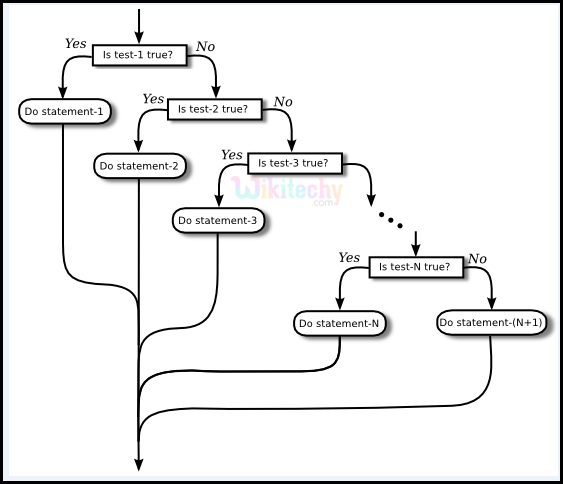Java If Statement

## Syntax :

```if (condition)
{
// code
if (condition) 		\\ nested
{		}
}
else if (condition)		\\ extended to next level
{
// code
}
else if (condition)		\\ extended to next level
{
// code
}
else
{
// code
}
```

## Sample Code :

```public class MyIfSample
{
public static void main(String args[])
{
System.out.println("\n\nWikiTechy - If Statement\n");
int a = 20, b = 30;
if(a > b)
{
System.out.println("Value of a is greater");
}
else if (b > a)
{
System.out.println("Value of b is greater");
}
else
System.out.println("Values of a and b are equal");
}
}
```

## Code Explanation :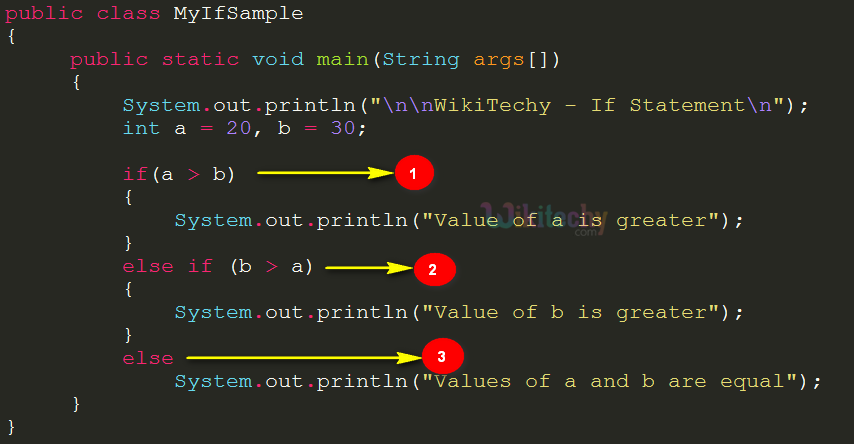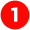If statement compares the value of variables a and b and returns result as a Boolean expression via the code if (a > b). If the condition is satisfied, then the output in the console will be as “Value of a is greater”.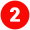Extension of the if statement is performed via the code else if (b > a). If the condition in the else if statement is satisfied, then the output will be as “Value of b is greater”.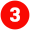The else statement is present here to deliver a default code to be executed if none of the if or else if expressions satisfies.

##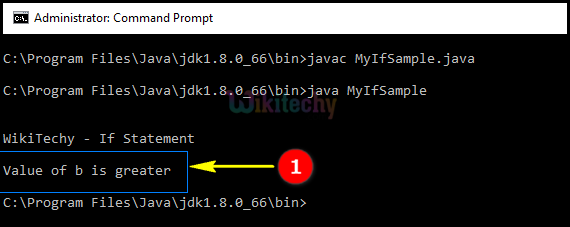Here in this java output, we have shown that the “WikiTechy – IF Statement“  executes the else if statement “ Value of b is greater “ where the value of b=30 is greater than  a=20 .

Bug Bounty
Webinar